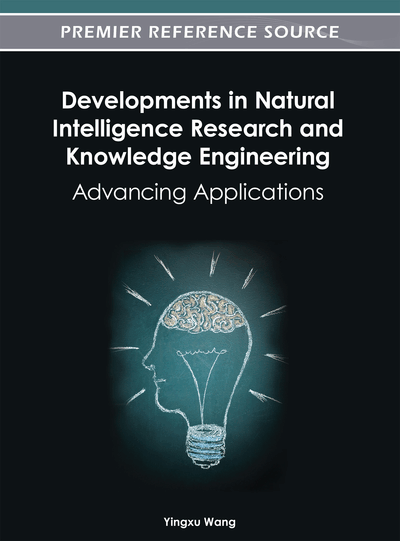# Generalized Rough Logics with Rough Algebraic Semantics

Jianhua Dai (College of Computer Science, Zhejiang University, China)
DOI: 10.4018/978-1-4666-1743-8.ch013

## Abstract

The collection of the rough set pairs of an approximation (U, R) can be made into a Stone algebra by defining two binary operators and one unary operator on the pairs. By introducing a more unary operator, one can get a regular double Stone algebra to describe the rough set pairs of an approximation space. Sequent calculi corresponding to the rough algebras, including rough Stone algebras, Stone algebras, rough double Stone algebras, and regular double Stone algebras are proposed in this paper. The sequent calculi are called rough Stone logic (RSL), Stone logic (SL), rough double Stone logic (RDSL), and double Stone Logic (DSL). The languages, axioms and rules are presented. The soundness and completeness of the logics are proved.
Chapter Preview
Top

## Introduction

Rough set theory was invented by Pawlak to account for the definability of a concept in terms of some elementary ones in an approximation space (U, R), where U is a set, and R is an equivalence relation on U. It captures and formalizes the basic phenomenon of information granulation. The finer the granulation is, the more concepts are definable in it. For those concepts not definable in an approximation space, the lower and upper approximations for them can be defined. These approximations construct a representation of the given concept in the approximation space.

Research on rough sets by algebraic method has gathered many researchers’ attention such as (Iwinski, 1987; Pomykala et al., 1988; Gehrke, 1992; Comer, 1993; Lin et al., 1994; Pagliani, 1996; Banerjee et al., 1996; Banerjee, 1997; Jarvinen, 2002; Dai, 2004; Dai, et al., 2006(a), Dai et al., 2006(b); Dai, 2007; Dai, 2008). In (Lin et al., 1994), the researchers studied the approximations under the structure of set algebra. Iwinski paid more attention about the lattice properties of set algebra and proposed the method of defining of rough approximations (Iwinski, 1987). Based on atomic Boolean lattice, Jarvinen proposed a novel definition method of rough approximations (Jarvinen, 2002). Based on the molecules, Dai constructed a more general structure of rough approximations based on molecular lattices (Dai 2004). At the same time, researchers also study rough sets from the point of description of the rough set pairs i.e. <lower approximation set, upper approximation set>. Pomykala and Pomykala laid a foundation for this field (Pomykala et al., 1988). They used Stone algebra to describe rough sets. They showed that collection of the rough set pairs of an approximation (U, R) can be made into a Stone algebra. By introducing another unary operator, Comer got a regular double Stone algebra to describe the rough set pairs of an approximation space (Comer, 1993). Pagliani adopted semi-simple Nelson algebra (Pagliani, 1996). Banerjee and Chakraborty defined pre-rough algebra which is more structured than topological quasi-Boolean algebra and used pre-rough algebra to describe rough sets (Banerjee et al., 1996). Some rough algebras with Brouwer-Zadeh lattices and 3-valued Lukasiewicz algebras were connected (Dai et al., 2006(a); Dai et al., 2006(b); Dai, 2007). Recently, the concept of rough 3-valued Lukasiewicz algebras was proposed and studied in (Dai, 2008).

## Complete Chapter List

Search this Book:
Reset